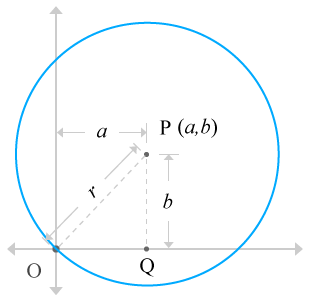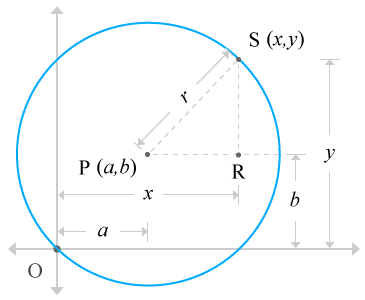# Equation of a Circle when the circle passes through the origin

Expressing a circle in mathematical equation form when the circle passes through the origin is defined equation of a circle when the circle passes through the origin.

A circle can be passed through the origin of the Cartesian coordinate system. It can be expressed in algebraic form, based on the geometrical relation between the circle and Cartesian coordinate system. Here, you learn how to develop an equation of a circle in algebraic form expression when the circle passes through the origin of the Cartesian coordinate geometric system.

## DerivationAssume, $O$ is an origin of the Cartesian coordinate system.

Assume, a circle having $r$ units radius, passes through the origin of the Cartesian coordinate geometric system.

Assume, the centre of the circle is located at $a$ units distance in horizontal direction and $b$ units distance in vertical direction from the origin. The centre of the circle is named $P$. So, the coordinates of the centre is represented as $P\left(a,b\right)$ in coordinate geometric system.

Join the origin $O$ and centre of the circle $P$. Actually, the circle is passing through the origin. Therefore, the distance from origin to centre of the circle is equal to the radius of the circle. So, $\stackrel{‾}{OP}=r$.

Draw a perpendicular line to horizontal axis from the centre of the circle and assume, it meets horizontal axis at a point, named $Q$. According to figure, the point $Q$ is located at $a$ units distance from the origin in horizontal axis direction and zero units distance from the same origin in vertical axis direction. Therefore, the distance between origin and point $Q$ is $\stackrel{‾}{OQ}=a$.

The distance from point $Q$ to centre of the circle is $\stackrel{‾}{PQ}=b$.

A right angle triangle, known $\Delta POQ$ is constructed inside the circle. The sides of the right angle triangle are line segments $\stackrel{‾}{OP},\stackrel{‾}{PQ}$ and $\stackrel{‾}{OQ}$.

According to the Pythagorean Theorem,

${\left(Hypotenuse\right)}^{2}={\left(Opposite side\right)}^{2}+{\left(Adjacent side\right)}^{2}$

${OP}^{2}={PQ}^{2}+{OQ}^{2}⇒{r}^{2}={b}^{2}+{a}^{2}$

Later, this mathematical relation will be used in developing the equation of the circle.

It will be used later in developing the equation of the circle. So, look away from this mathematical relation temporarily.Consider a point on the circle and assume the point represents each and every point on the circle. It means, it is a variable point, named $S$. Assume, it is located at $x$ units distance in horizontal axis direction and $y$ units distance in vertical axis direction from the origin. Therefore, the coordinates of the point $S$ is written $S\left(x,y\right)$.

Join the point $P$ and $S$ and the distance between point $P$ and $S$ is equal to radius of the circle. Therefore, $\stackrel{‾}{PS}=r$.

Draw a perpendicular line towards horizontal axis from the point $S$ and also draw a line, parallel to the horizontal axis from point $P$. Assume, both lines meet at a point $R$. Thus, a right angled triangle, known $\Delta SPR$ is constructed.

Apply Pythagorean Theorem to right angled triangle $\Delta SPR$.

${PS}^{2}={SR}^{2}+{PR}^{2}$

As per the right angled triangle, and $\stackrel{‾}{PS}=r$. Substitute these values in the Pythagorean Theorem to get the required equation.

It is already proved above that ${r}^{2}={b}^{2}+{a}^{2}$. Substitute it in the above expression to start simplifying the expression.

It can be written as follows

$⇒{x}^{2}+{y}^{2}=2\left(ax+by\right)$

It is an algebraic equation which represents a circle when the circle passes through the origin of the Cartesian coordinate system.

Latest Math Topics
Latest Math Problems
##### Recommended Math Concepts###### How to develop equation of a Circle when the Circle touches x-axis###### How to derive equation of a Circle when the Circle touches y-axis###### How to develop equation of a Circle when the Circle touches both axes###### How to derive Equation of the Circle when centre of the circle coincides with the origin###### How to develop Equation of the Circle when Circle passes through the origin and Centre of the Circle lies on x-axis###### How to derive Equation of a Circle when Circle passes through the origin and Centre of the Circle lies on y-axis###### How to develop Equation of the Circle when Circle doesn’t passes through the origin and Centre of the Circle lies on x-axis###### How to develop Equation of the Circle when Circle doesn’t passes through the origin and Centre of the Circle lies on y-axis###### How to develop Equation of a Circle in Standard form

A best free mathematics education website for students, teachers and researchers.

###### Maths Topics

Learn each topic of the mathematics easily with understandable proofs and visual animation graphics.

###### Maths Problems

Learn how to solve the maths problems in different methods with understandable steps.

Learn solutions

###### Subscribe us

You can get the latest updates from us by following to our official page of Math Doubts in one of your favourite social media sites.